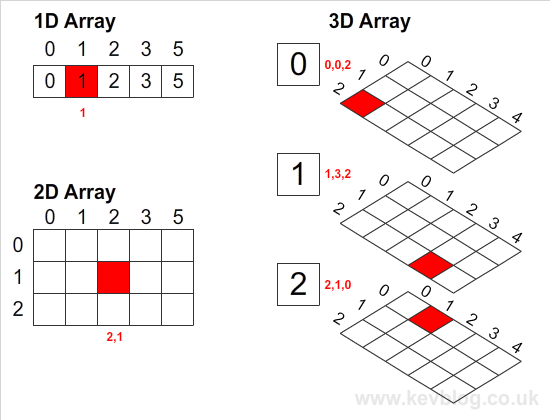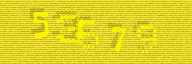# Create 3D Array in Actionscript 3

Thursday 10 Feb 2011 22:12

Creating a 2D or 3D array in Actionscript 3 is not something you can do natively. It is however very easy to do programmatically and on this page I'll explain how to do this easily. There's also a handy diagram to help visualise arrays with 1, 2 and 3 dimensions. Let's start with the code to create an array.

``` var arr:Array = new Array(5); ```

This code creates an array with space for 5 values. This array can be referenced like so:

``` arr = 3; trace(arr); // outputs 3 ```

If we want to create a 2 dimensional array, we can assign arrays to each of these 5 values like so:

``` var arr2D:Array = new Array(5); var x:int; for(x=0; x<5; x++) arr2D[x] = new Array(3); ```

This makes a 2D dimension array of size 5 x 3 which can be referenced like so:

``` arr2D = 6; trace(arr2D); // outputs 6 ```

2D arrays can be thought of as points on a 2D graph, the first index (2 above) being the x value and the second index (3 above) being the y value. 2D arrays are good for game maps such as a chess board or block puzzle game.

3D arrays are just a step on from the above. Imagine a series of 2D arrays stacked below each other (see my final diagram). This would create a 3 dimensional array. 3D arrays are useful for 3D games or maps that have a height to them. Taking the example to the next step we will create a base 3D array and to each element in that array, assign a 2D array like so:

``` var arr3D:Array = new Array(3); var z:int, x:int; for (z=0; z<3; z++) { arr3D[z] = new Array(5); for (x=0; x5; x++) arr3D[z][x] = new Array(3); } ```

We now have a 3D array dimensions 3 x 5 x 3. You could think of this as 3 layers of 5 x 3. The array can be referenced as: arr3D[z][x][y], where z is the layer and x and y are the 2D positions on that layer. I find it easiest to think of 3D arrays in these terms rarther than x,y and z. My final diagram visualises this and shows sample points (the red squares) in 1,2 and 3 dimesional arrays.